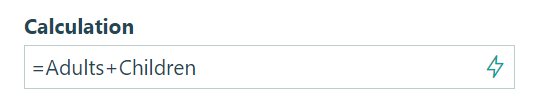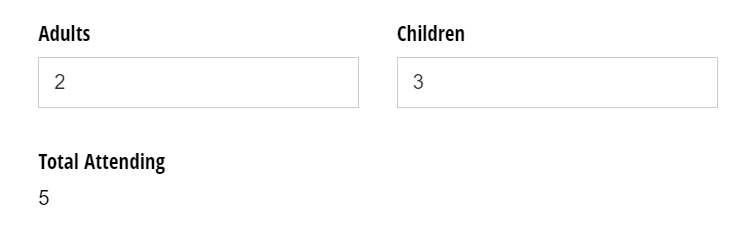# Numeric calculations

Cognito Forms makes it easy to write calculations that work with numbers. Start by using the appropriate field types to represent numbers: including Number, Currency, and Calculation (Number, Percent & Currency) fields.

## Syntax

For example, say you have two Number fields on your form, Adults and Children, representing the number of adults and children registering for an event. You could add a Calculation field that shows the total number of people attending the event, using the simple calculation: `=Adults + Children`Now, on your form, you can see this calculation in action:Here are examples of basic numeric calculations you could perform with these fields:

Type Example Results for 2 Adults and 3 Children
Addition `=Adults + Children` 2 + 3 = 5
Subtraction `=Adults - Children` 2 - 3 = -1
Multiplication `=Adults * Children` 2 * 3 = 6
Division `=Adults / Children` 2 / 3 = 0.66667
Equals `=Adults = Children`, or `=Adults == Children` 2 = 3 = false
Not Equals `=Adults != Children`, or `=Adults <> Children` 2 != 3 = true
Greater Than `=Adults > Children` 2 > 3 = false
Greater Than or Equals `=Adults >= Children` 2 >= 3 = false
Less Than `=Adults < Children` 2 < 3 = true
Less Than or Equals `=Adults <= Children` 2 <= 3 = true

When performing basic mathematical calculations, Cognito will treat blank values as zeros, so for example, 2 + blank would equal 2, and 2 > blank would equal true.

Additionally, you can perform more complex mathematical calculations by calling functions starting with Math, including important functions like Min, Max, Ceiling, Floor and Round. For example, you could write the calculation = Math.Max(Adults, Children) to calculate the maximum of these two values.

## Functions

Quick Tip

Select the function name from the list below to see an example of the function in action!

Here is a full list of supported math functions, which all return numbers:

Function Description
Math.Abs(Number) Returns the absolute value of the specified number.
Math.Acos(Number) Returns the angle whose cosine is the specified number.
Math.Asin(Number) Returns the angle whose sine is the specified number.
Math.Atan(Number) Returns the angle whose tangent is the specified number.
Math.Atan2(Number, Number) Returns the angle whose tangent is the quotient of the specified numbers.
Math.Ceiling(Number) Returns the smallest integer value that is greater than or equal to the specified number.
Math.Cos(Number) Returns the cosine of the specified angle.
Math.Cosh(Number) Returns the hyperbolic cosine of the specified angle.
Math.E Returns the natural logarithmic base.
Math.Exp(Number) Returns e raised to the specified power.
Math.Floor(Number) Returns the largest integer value that is less than or equal to the specified number. (Round down)
Math.Log(Number) Returns the natural logarithm of the specified number.
Math.Log(Number, Number) Returns the logarithm of arg1 in the base arg2. i.e. Log(num, base)
Math.Log10(Number) Returns the base 10 logarithm of the specified number.
Math.Max(Number, Number) Returns the larger of the two specified numbers.
Math.Min(Number, Number) Returns the smaller of the two specified numbers.
Math.PI Returns the ratio of the circumference of a circle to its diameter.
Math.Pow(Number, Number) Returns the number arg1 raised to the power arg2. i.e. Pow(num, power)
Math.Round(Number) Returns the nearest integer value. We use bankers’ rounding to round values to the closest even integer (ex: 1.5 rounds to 2).
Math.Round(Number, Number) Returns a decimal value to a specified number of fractional digits. We only support displaying up to 2 fractional digits. i.e. Round(num, fracDigits). The number of fractional digits must be a positive number between 0 and 28.
Math.Sign(Number) Returns a value representing the sign of the specified number. Returns -1, 0, or 1 which means the number is negative, 0, or positive, respectively.
Math.Sin(Number) Returns the sine of the specified angle.
Math.Sinh(Number) Returns the hyperbolic sine of the specified angle.
Math.Sqrt(Number) Returns the square root of the specified number.
Math.Tan(Number) Returns the tangent of the specified angle.
Math.Tanh(Number) Returns the hyperbolic tangent of the specified angle.
Math.Truncate(Number) Returns the integral part of the specified number.
% Modulus Returns the remainder that results from performing integer division (also known as the modulus function).

## Number formats

After inserting a Number field on your form, you can customize the format of the number as it appears on other parts of your form. To do this, simply enter a formatted string into a calculation field.

### Number

Format a number, without thousand separators, with the specified number of decimal places.

Function Returns
`=Form.Number.ToString("N")` 123.46
`=Form.Number.ToString("N0")` 123
`=Form.Number.ToString("N1")` 123.5
`=Form.Number.ToString("N2")` 123.46
`=Form.Number.ToString("N3")` 123.456
`=Form.Number.ToString("N10")` 123.4560000000

### Digits

Format a number with the minimum specified number of digits, padding positive numbers with leading zeros if necessary.

Function Returns
`=Form.Number.ToString("D")` 1
`=Form.Number.ToString("D1")` 1
`=Form.Number.ToString("D2")` 01
`=Form.Number.ToString("D3")` 001
`=Form.Number.ToString("D10")` 0000000001

### Currency

Format a number as a currency value, with currency symbol, thousands separators, parenthesis around negative numbers, and the specified number of decimal places.

Function Returns
`=Form.Number.ToString("C")` \$123.46
`=Form.Number.ToString("C0")` \$123
`=Form.Number.ToString("C1")` \$123.5
`=Form.Number.ToString("C2")` \$123.46
`=Form.Number.ToString("C3")` \$123.456
`=Form.Number.ToString("C10")` \$123.4560000000

### Percentage

Format a number multiplied by 100 with a percentage symbol, with the specified number of decimal places.

Function Returns
`=Form.Number.ToString("P")` 12,345.60%
`=Form.Number.ToString("P0")` 12,346%
`=Form.Number.ToString("P1")` 12,345.6%
`=Form.Number.ToString("P2")` 12,345.60%
`=Form.Number.ToString("P3")` 12,345.600%
`=Form.Number.ToString("P10")` 12,345.6000000000%